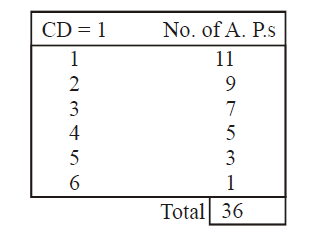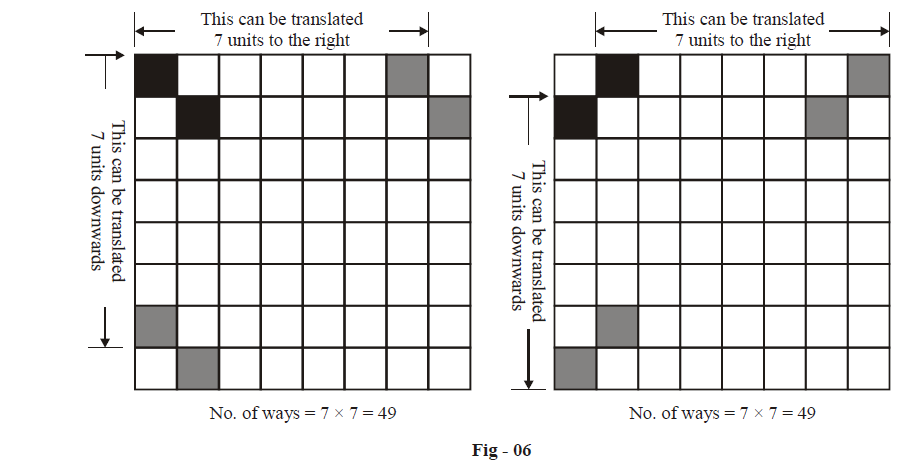# Examples on Probability Set 2

Go back to  'Probability'

Example – 6

Consider the sequence of numbers 1, 2, 3, ...., 13. A person chooses three numbers at random from this sequence. Find the probability that the three numbers form an A.P.

Solution: Note that A. P. s can be formed with varying common differences (CD). (1, 2, 3) and (3, 4, 5) are examples of A. P. s with CD 1. (2, 5, 8) is an A. P of CD 3. The maximum CD possible is 6, in the A. P. (1, 7, 13).

Let us count all such A. P.s in a table. Verify the column on the right.Thus, the total number of APs possible from this set is 36. Also, from this set of 13 numbers, a selection of 3 numbers can be made in $$^{13}{{C}_{3}}$$= 286 ways.

Therefore, the probability that three numbers picked at random from this set form an A.P. is \begin{align}\frac{36}{286}=\frac{18}{143}\end{align} .

Example – 7

A pair of dice is rolled until a sum of either 5 or 7 is obtained. Find the probability that 5 comes before 7.

Solution: A sum of 5 can be obtained in 4 ways, namely

$\{(1,\ 4)\ (2,\ 3)\ (3,\ 2)\ (4,\ 1)\}$

from a total number of 36 ways of throwing a pair of dice. If we let E denote the event 5 of obtaining a sum of 5, we have

$P(E)=\frac{4}{36}=\frac{1}{9}$

Similarly, let the event F be that of obtaining a sum of 7; this can happen in 6 ways, namely

$\left\{ (1,\ 6)\ (2,\ 5)\ (3,\ 4)\ (4,\ 3)\ (5,\ 2)\ (6,\ 1) \right\}$

so that

$P(F)=\frac{6}{36}=\frac{1}{6}$

Finally, if we let G be the event of obtaining neither a 5 or a 7, we have

\begin{align}& P(G)=1-P(E)-P(F) \\ & \quad\quad\;=1-\frac{1}{9}-\frac{1}{6} \\ & \quad\quad\;=\frac{13}{18} \\ \end{align}

Now, we want a sum of 5 to come before a sum of 7. Think about how this can happen. Every time you roll the pair of dice, you should either get a sum of 5 or you should get neither a sum of 5 nor 7.

Therefore, the following (mutually exclusive) sequences of throws leads us to a sum of 5 before a sum of 7.

 Sequence P(Sequence) E \begin{align}\frac{1}{9}\end{align} G, then E \begin{align}\frac{13}{18}\times \frac{1}{9}\quad\text{(Successive Rolls are independent events!)}\end{align} G, then G, then E \begin{align}\frac{13}{18}\times \frac{13}{18}\times \frac{1}{9}\end{align} G, then G, then G, then E \begin{align}\frac{13}{18}\times \frac{13}{18}\times \frac{13}{18}\times \frac{1}{9}\end{align} $\vdots$ $\vdots$

The required probability is obtained by adding the terms in the right column.

\begin{align}& P\left( 5\text{before}\text{ }7 \right)\text{ }=\frac{1}{9}+\frac{13}{18}\ \cdot \ \frac{1}{9}+{{\left( \frac{13}{18} \right)}^{2}}\ \cdot \ \frac{1}{9}+{{\left( \frac{13}{18} \right)}^{3}}\ \cdot \ \frac{1}{9}+\ .....\infty \\ &\qquad\qquad\quad\quad\; =\frac{1}{9}\left( 1+\frac{13}{18}\ +\ {{\left( \frac{13}{18} \right)}^{2}}\ +{{\left( \frac{13}{18} \right)}^{3}}+\ .....\infty \right) \\ &\qquad\qquad\quad\quad\; =\frac{1}{9}\ \cdot \ \frac{1}{1-\frac{13}{18}} \\ &\qquad\qquad\quad\quad\; =\frac{2}{5} \\ \end{align}

In passing, note that the probability of obtaining a 7 before 5 is simply

\begin{align}& P\left( 7\text{ before }5 \right)\text{ }=\text{ }1-\text{ }P\left( 5\text{ before }7 \right) \\ &\qquad\qquad\qquad \;=\frac{3}{5} \\ \end{align}

Can you appreciate why obtaining a 7 before 5 is more likely than obtaining a 5 before 7?

Example – 8

Two unit squares are chosen at random from a standard chessboard. What is the probability that the two squares have exactly one corner in common?

Solution: The total number of ways of selecting two squares from a standard chessboard is simply $$^{64}{{C}_{2}}\text{ }=\text{ }2016$$.

Now, let us find the number of ways of selecting a pair with exactly one corner in common. For that, consider the following figure.It is immediately evident that the number of favorable ways is 49 + 49 = 98.

The required probability is \begin{align}\frac{98}{2016}=\frac{7}{144}\end{align}

Example – 9

For two events A and B, we are given the following information:

$P(A)=\frac{1}{4},\ \ P(A/B)=\frac{1}{4}\ \ \text{and}\ \ P(B/A)=\frac{1}{2}$

(a) Determine whether the two events are ME or independent, or neither.

(b) Find $$P(\bar{B}/\bar{A})$$ . The notation  $$\bar{A}$$ stands for the complementary event of event A, i.e., $$\bar{A}$$ occurs if A does not occur  $$(\bar{A}=not-A)$$.

Solution: (a) Since P(A) and P(A / B) are the same, this means that A and B are independent events. Hence they are obviously not ME.

We therefore obtain

\begin{align}&\quad P(A\cap B)=P(A)\cdot P(B)\qquad\left( independence \right) \\ &\; \Rightarrow\quad P(B)=\frac{1}{2} \\ \end{align}

(b) Now, if A and B are independent events, then so are $$\bar{A}\,\,and\,\,\bar{B}$$  (and in fact (A and  $$\bar{B}$$) and  $$(\bar{A}\ \,\text{and}\,B)$$ . This fact should be intuitively obvious but let us justify it with some rigor. We have

\begin{align} &\qquad \qquad\bar{A}\cap \bar{B}=(\overline{A\cup B})\left\{ \begin{gathered} \text{From}\,\text{elementary set theory; try to } \\\\ \text{justify this using a venn diagram} \\\\ \end{gathered} \right\} \\\\ & \Rightarrow \quad P(\bar{A}\cap \bar{B})=P(\overline{A\cup B}) \\\\ &\qquad\qquad\quad\quad\;\; =1-P(A\cap B) \\ \\ &\qquad\qquad\quad\quad\;\; =1-\left\{ P(A)+P(B)-P(A\cap B) \right\} \\\\ &\qquad\qquad\quad\quad\;\; =1-P(A)-P(B)+P(A)\cdot P(B)\left\{ \text{Since A, B are independent }\right\} \\\\ & \qquad\qquad\quad\quad\;\; =(1-P(A))\ (1-P(B)) \\\\ &\qquad\qquad\quad\quad\;\; =P(\bar{A})\ \cdot \ P(\bar{B}) \\\\ &\qquad\qquad\quad\;\; \Rightarrow\quad \bar{A}\ \ \text{and}\ \ \bar{B}\,\,\,are\;\text{independent } \\ \end{align}

Similar proofs follow for the other two pairs.

Returning to the question, we see that since  $$\overline{A}\,\,and\,\,\overline{B}$$ are independent, we have

$P(\bar{B}/\bar{A})=P(\bar{B})=1-P(B)=\frac{1}{2}$

Example – 10

In this example, we’ll get a taste of what is known as inverse probability, which we’ll of course be discussing in detail in a later section.

A man is known to speak the truth 3 out of 4 times. He throws a die and tells you that he obtained a six. What is the chance of it being really a six ?

Solution: The problem here is that some event has already taken place, i.e., the throwing of a die. What we are required to do is find out how truthful the result being told to us is, given that the person reporting the result is not exactly a saint!

So, we first let E, F, G denote the following events:

E : Man reports a six

F : Die shows a six

G : Die does not show a six

Observe that we are required to find P(F / E), i.e., the probability of the die actually showing a six given that the man is reporting so.

Since we already know a bit about conditional probabilities, let us try to follow that route of manipulation.

$P(F/E)=\frac{P(F\cap E)}{P(E)}=\frac{P(F)P(E/F)}{P(E)}$

Now, $$F\cap E$$ is the event that the man reports a six when actually he obtains a six. The probability of this event will simply be

\begin{align} & P(F\cap E)=P(F)\ \cdot \ P(E/F) \\ &\qquad\qquad =\frac{1}{6}\ \cdot \ \frac{3}{4} \\ & \qquad\qquad=\frac{1}{8} \\ \end{align}

You are urged to carefully understand how we arrived at this.

Next, we try to find P(E), i.e., the probability of the man reporting a six. This can happen in two mutually exclusive ways.

 Way P (Way) Actual result : Six Man says the truth $\frac{1}{6}\,\,\times \,\,\frac{3}{4}\,\,=\,\,\frac{1}{8}$ Actual result : Not a six      Man lies $\frac{5}{6}\,\,\times \,\,\frac{1}{4}\,\,=\,\,\frac{5}{24}$

\begin{align}Thus,\qquad\qquad & P(E)=\frac{1}{8}+\frac{5}{24} \\ & \qquad\;=\frac{1}{3} \\ \end{align}

\begin{align}& Finally,\qquad\qquad P(F/E)=\frac{1/8}{1/3} \\ &\qquad\qquad\qquad\qquad\qquad\quad\;=\frac{3}{8} \\ \end{align}

## TRY YOURSELF - I

It should be remarked that a lot of readers might not understand fully the underlying idea of this problem. They need not despair since inverse probabilities will be dealt with in much detail later.

Q. 1 Generalise the result of example - 6 by considering the sequence of (2n + 1) numbers : 1, 2, 3, ..., (2n + 1).

Q. 2 A man and a woman appear in an interview for two vacancies for the same post. Their respective probalilities of selection are  \begin{align}\frac{1}{4}\,and\,\frac{1}{3}\end{align}. Find the probability that

(a) at least one of them will be selected

(b) none of them will be selected.

Q. 3 If \begin{align}\frac{1+3p}{3},\ \ \frac{1-p}{4}\ \ \text{and}\ \ \frac{1-2p}{2}\end{align} are the probabilities of three mutually exclusive events, then show that  \begin{align}p\in \left[ \frac{1}{3},\,\,\frac{1}{2} \right]\end{align}.

Q. 4 A, B and C are three mutually independent events. Prove that

(a) A and $$(B\cap C)$$ are independent

(b) A and $$(B\cup C)$$ are independent

Q. 5 A box contains 2 black, 4 white and 3 red balls. One ball is drawn at random from the box and kept aside. From the remaining balls in the box another ball is drawn at random and kept besides the first. This process is continued till all the balls are drawn. Find the probability that the balls drawn are in the sequence of 2 black, 4 white and 3 red balls.

Q. 6 The probability of a man hitting a target in one fire is \begin{align}\frac{1}{4}\end{align} . At least how many times must he fire at the target so that the chance of his hitting the target at least once is greater than \begin{align}\frac{2}{3}\end{align} .

Q. 7 Two squares are chosen at random on a chessboard. Find the probability that they have no side in common.

Q. 8 Four people A, B, C, D are playing a game: each person draws a card from a standard well-shuffled deck, puts the card back, shuffles the deck and passes if to the next person. The game starts with A and follows the order  $$A\xrightarrow{{}}B\xrightarrow{{}}C\xrightarrow{{}}D$$  and then back again. The person who draws a spades first wins. Find their respective chances of winning the game.

Q. 9 There are n persons seated around a circular table, from which two are selected at random. Find the probability that the two persons are not adjacent.

Q. 10 n positive integers are taken at random and multiplied. Find the probability that the last digit of the product is 2, 4, 6 or 8.